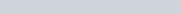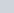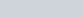ChemTalk

# Calorimetry## Core Concepts

In this article, you will learn about calorimetry and calorimeters, mainly used to calculate specific heat capacity and thermal changes in a physical or chemical reaction. Concepts like different types of calorimeters and the calorimeter equation will also be covered.

## What is Calorimetry?

Calorimetry is a field of thermochemistry that measures the amount of heat involved in a physical or chemical reaction. Chemists use calorimetry to determine the amount of heat transferred to or from a system into its surroundings. From a chemical reaction like acid-base neutralisation to a physical reaction like solidifying, calorimetry allows scientists to measure thermal changes within any reaction.

To measure heat change, chemists first define the reaction’s system vs. surroundings. In thermodynamics, a system is the substance/substances undergoing the chemical or physical reaction, while the surroundings is all other matter. The surroundings serve to either provide or absorb heat to the system. The temperature difference between surroundings and system is then used to derive the amount of heat transferred by the reaction.

## What is a Calorimeter?

Crucial to calorimetry, a calorimeter is any device used to measure the amount of heat involved in a surroundings-system transfer. The change in temperature can be used with specific heat and mass to determine the amount of heat involved in a reaction.

A calorimeter can also be used to determine the specific heat capacity of certain substances involved in the heat transfer reaction.

There are many different types of calorimeters that accomplish specific functions and measure certain qualities of a substance. However, most calorimeters consist of a metallic vessel which is well-insulated to prevent additional energy from escaping into the surroundings.

This vessel’s only opening is for the thermometer to measure changes in the reaction’s thermal properties. This thermometer measures significant changes in heat, allowing chemists to determine heat capacities and the total energy used in the reaction.

## Exothermic vs. Endothermic Reactions

An endothermic reaction is when the system or reaction gains energy from its surroundings. For example, dissolving a solution of ammonium nitrate in water is an endothermic chemical process because the water loses thermal energy (which explains how instant cold packs work)!

On the other hand, endothermic reactions can be explained by the equation below, where q represents heat. Notice that the system has a positive q-value, indicating that the system gained heat through the reaction. On the other hand, the surroundings has a negative q-value because the surroundings lost heat to the system.Exothermic reactions involve the system releasing energy into the surroundings – any chemical reaction that produces heat, like an instant hot pack, is an exothermic reaction. In these reactions, the system loses energy while the surroundings gain energy, as represented by the equation below.Moreover, the amount of energy transferred from systems to surroundings must always equal each other. Therefore, this fundamental phenomenon abides by the First Law of Thermodynamics – which states that energy can never be destroyed or created in a reaction.

## Calorimetry Equation

When analysing a heat transfer reaction, chemists use the calorimetry equation relating heat released in the reaction to the substance’s mass, change in temperature, and specific heat capacity.

In the equations, q is the amount of heat measured in joules. m is mass measured in grams.as change in temperature measured in Celsius, or the initial temperature subtracted from the final temperature. c is the specific heat capacity of a substance, or the amount of joules needed to raise the temperature by 1 degree Celsius.

When provided with either of these values, one can easily calculate any other value in the equation and determine what sign the q-value is (indicating whether the reaction is exothermic or endothermic).## Types of Calorimetry

Moreover, calorimetry falls under three main categories, based on which factors remain constant: constant pressure calorimetry, constant volume calorimetry and differential scanning calorimetry.

1. Constant Pressure Calorimetry

By keeping external vs. internal pressure consistent, this calorimetry isolates the systems from losing or gaining heat from surroundings. Chemistsuse a coffee cup-like device in this type of calorimetry, which merely insulates the system.

2. Constant Volume Calorimetry

Similar to its name, constant volume calorimetry aims to maintain a constant volume and resist large amounts of pressure. This leads to higher accuracy when calculating heat transfer and this calorimeter is known as a “bomb calorimeter,” a device that relates heat of reaction and specific heat capacity.

3. Differential Scanning Calorimetry

Including both a sample and a reference chamber, differential scanning calorimetry compares specific heat capacities of the same solvent. The sample and reference chambers both contain the same volumes of solvent, while the sample chamber also contains the substance of interest. Any changes in the heat of reaction throughout the reaction can thus be attributed to the interested substance, which is impacting the calculated heat capacity.

## Calorimetry Practice Problems

1. When 1g of glucose is combusted in a bomb calorimeter, the temperature of the calorimeter increases by 2 degrees C. The heat capacity of the calorimeter and its contents is 9.90 kJ/degrees C. What is the q-value for this combustion?
2. How many mL of water at 23 °C with a density of 1.00 g/mL must you mix with 180 mL of coffee at 95 °C so that the resulting combination will have a temperature of 60 °C? Assume that coffee and water have the same specific heat (4.184 J/g °C).
3. You place a 45-g aluminium spoon (specific heat 0.88 J/g °C) at 24 °C in 180 mL (180 g) of coffee at 85 °C. The temperature of the two become equal.
4. The temperature of the cooling water as it leaves the hot engine of an automobile is 240 °F. After it passes through the radiator it has a temperature of 175 °F. Calculate the amount of heat transferred from the engine to the surroundings by one gallon of water with a specific heat of 4.184 J/g °C.
5. How much will the temperature of a cup (180 g) of coffee at 95 °C decrease when a 45 g silver spoon (specific heat 0.24 J/g °C) at 25 °C enters the coffee and the two reach the same temperature? Assume that the coffee has the same density and specific heat as water.

1. q = 19.8 kJ
2. 170 mL
3. 81.95 °C
4. 570 kJ
5. 1 degree